Do you like the article?
Share it with others -

Use new possibilities of MetaTrader 5

# One-Minute Data Modelling Quality Rating

16 June 2006, 11:19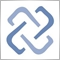0
5 309

The formula of modelling quality calculation is given in the article named What the Numbers in the Expert Testing Report Mean:
```ModellingQuality = ((0.25*(StartGen-StartBar) +
0.5 *(StartGenM1-StartGen) +
0.9 *(HistoryTotal-StartGenM1)) / (HistoryTotal-StartBar))*100%;```
where:
• HistoryTotal - the total amount of bars in history;

• StartBar - the number of bar with which the testing was started. Modeling starts at at least 101st bar or the bar corresponding with the initial date of test limits;

• StartGen - the number of bar with which the modeling on the nearest timeframe started;

• StartGenM1 - the number of bar with which the modeling on minutes started;

at that:

• the distance between the beginning of modeling of databases for the nearest timeframe and the beginning of modeling on the nearest timeframe data has a weighting factor of 0.25;

• the distance between the beginning of modeling on the nearest timeframe data and the beginning of modeling on minutes has a weighting factor of 0.5;

• the distance between the beginning of modeling on minutes and the end of history data has a weighting factor of 0.9.

Data modelled on one-minute timeframes are rated very high: 90% of quality.

Reasoning from the same formula, the one-minute data modelled cannot be of quality exceeding 25% since data of a smaller period are not used for modelling of these data. For client terminal, there are simply no data of a smaller period, but there are tick data. However, tick data are not stored in the client terminal by standard means.

In themselves, one-minute data are the most detailed data about the price movement. And, using them, one can model the price movement inside of bars of larger periods. At that, discrepancy between the real price movement and the ticks modelled will not be large due to presence of one-minute reference points: Open, High, Low, Close. These reference points form the first approximation of the price movement function (the first-order approximation). The higher is the value of the period modelled, the more there are reference points and the more exact is the modelling. And vice-versa, the lower the value of the modelled period is, the lower is the modelling quality. For example, up to 240 one-minute reference points can be used to model a one-hour bar. When modelling a 5-minute bar, the amount of reference points drops to 20.

As we mentioned before, there are just 4 reference points for a one-minute bar to be formed! This is why the rating of modelling quality for one-minute bars does not exceed 25%. However, if one uses signals received from data of a larger timeframe when testing on one-minute timeframe, the data modelled should, of course, be rated much higher because the price movement within the bar of timeframe larger than the on-minute one is considered.

Note: Strictly speaking, data modelled on one-minute base should come within definition of "modelling on base of history data of the nearest timeframe" at calculation of 5-minute modelling quality and, respectively, be rated at 0.5 and not 0.9. However, we decided not to introduce an additional formula and complicate the modelling algorithm in this way.

Translated from Russian by MetaQuotes Software Corp.
Original article: https://www.mql5.com/ru/articles/1513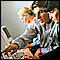Genetic Algorithms: Mathematics

Genetic (evolutionary) algorithms are used for optimization purposes. An example of such purpose can be neuronet learning, i.e., selection of such weight values that allow reaching the minimum error. At this, the genetic algorithm is based on the random search method.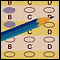How to Evaluate the Expert Testing Results

The article gives formulas and the calculation order for data shown in the Tester report.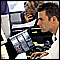Free-of-Holes Charts

The article deals with realization of charts without skipped bars.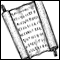Information Storage and View

The article deals with convenient and efficient methods of information storage and viewing. Alternatives to the terminal standard log file and the Comment() function are considered here.